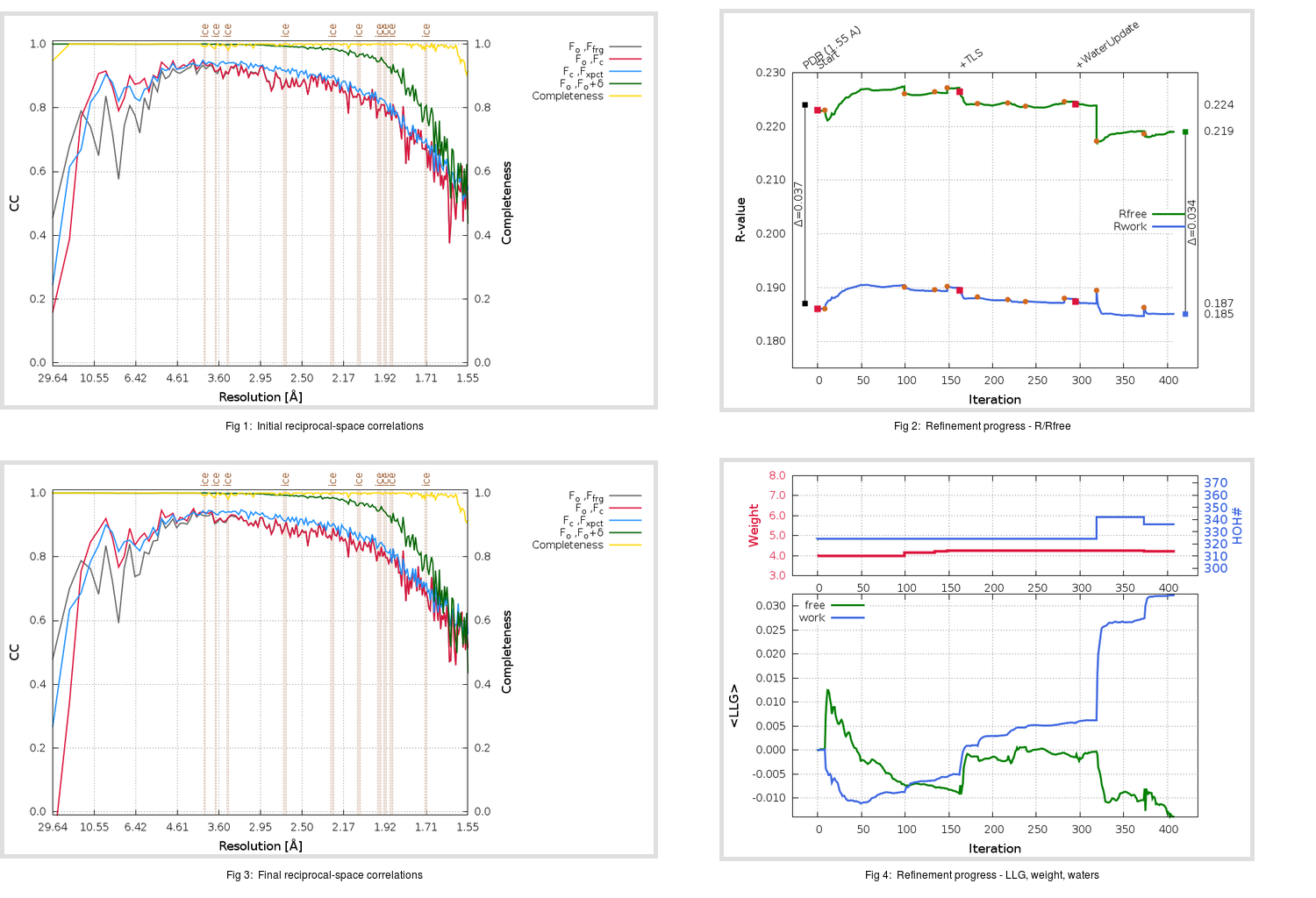Content:

```    Diffraction limits & principal axes of ellipsoid fitted to diffraction cut-off surface:
1.737         0.9350   0.0000  -0.3548       0.973 a* - 0.230 c*
1.488         0.0000   1.0000   0.0000       b*
1.553         0.3548   0.0000   0.9350       0.731 a* + 0.682 c*
```

## Deposited

` `
 Date deposited Date data collection Resolution R, Rfree 20200315 20200304 1.55 0.1850 0.2240

Molprobity (CCP4 7.0 version) summary:

```Ramachandran outliers =   0.33 %
favored =  98.01 %
Rotamer outliers      =   1.14 %
C-beta deviations     =     0
Clashscore            =   4.44
RMS(bonds)            =   0.0134
RMS(angles)           =   1.83
MolProbity score      =   1.26
Resolution            =   1.55
R-work                =   0.1850
R-free                =   0.2240
```

```Number of waters      =   324

<B> (all atoms) =   22.01 ( sd =    8.80 ) for       2722 non-hydrogen atoms
<B>   (protein) =   20.59 ( sd =    7.85 ) for       2370 non-hydrogen atoms
<B>     (water) =   31.92 ( sd =    8.80 ) for        324 non-hydrogen atoms
<B>    (others) =   28.30 ( sd =   10.91 ) for         28 non-hydrogen atoms

B min/max       (all non-hydrogen atoms) =   10.32 /   67.41
B min/max   (protein non-hydrogen atoms) =   10.71 /   67.41
B min/max     (water non-hydrogen atoms) =   10.32 /   57.94
B min/max     (other non-hydrogen atoms) =   20.17 /   34.83
```

## BUSTER (re-)refinement

` `

Molprobity (CCP4 7.0 version) summary:

```Ramachandran outliers =   0.33 %
favored =  98.34 %
Rotamer outliers      =   1.14 %
C-beta deviations     =     0
Clashscore            =   2.32
RMS(bonds)            =   0.0115
RMS(angles)           =   1.56
MolProbity score      =   1.05
Resolution            =   1.55
R-work                =   0.1851
R-free                =   0.2191
```

```Number of waters      =   336

<B> (all atoms) =   23.20 ( sd =    8.58 ) for       2734 non-hydrogen atoms
<B>   (protein) =   21.47 ( sd =    6.84 ) for       2370 non-hydrogen atoms
<B>     (water) =   34.91 ( sd =   10.03 ) for        336 non-hydrogen atoms
<B>    (others) =   29.10 ( sd =   11.28 ) for         28 non-hydrogen atoms

B min/max       (all non-hydrogen atoms) =    6.58 /   68.83
B min/max   (protein non-hydrogen atoms) =    6.58 /   62.65
B min/max     (water non-hydrogen atoms) =   12.07 /   68.83
B min/max     (other non-hydrogen atoms) =   22.42 /   35.09
```

Refinement progression:Results:

` `
 File Remark 5REW_aB_refine.01_03_refine.pdb.gz exact refinement commands are in header 5REW_aB_refine.01_03_refine.mtz.gz including original deposited data and several re-refinement map coefficients 5REW_aB_refine.01_03_BUSTER_model.cif.gz including any non-standard compound restraints 5REW_aB_refine.01_03_BUSTER_refln.cif.gz